Comparing the Approximation to the Exact Solution

The exact minimizing path can be found by using Calculus of Variations (demonstrated below). The solution is obtained from a boundary-value problem which we do not take up at this point, but it is interesting to see the exact solution and compare it with the approximate one we obtained above.  The closed-form expression for the function that minimizes the climbing time is: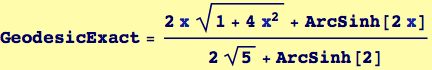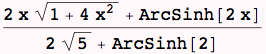Graphical Comparisons

Plot the exact solution and compare it to our calculated approximation...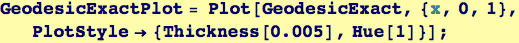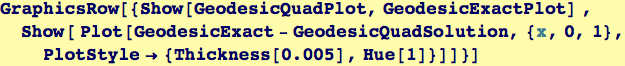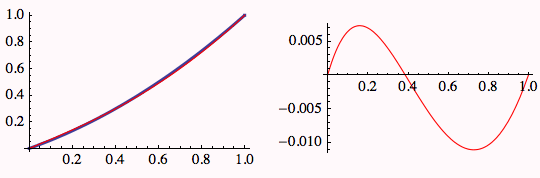These two curves are very close, comparinfg three distances: the quadratic approximation, the exact solution, and a straight line.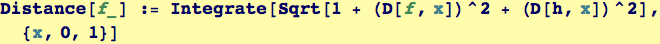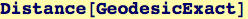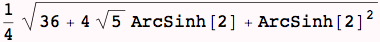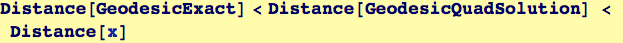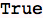Created by Wolfram Mathematica 6.0  (26 November 2007)